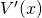# Volume of Revolution Discussion iii

## Let’s recap:

So far we have seen that the volume of revolution formula using calculus applied to a graph such asagrees with the volume of a cylinder formula.

We have also seen that there exists a volume function which maps the volume at a certain value, and refers to the volume of the solid generated by rotating a function around theaxis 360°.

Here we see that if our functionis smooth and continuous, our functionwill be smooth and continuous.

The function f(x) is drawn on the 3D graph on the left hand side. Slide the value(slowly) to see the function, the volume, plotted on the xy plane on the right hand side.

You may change the function to any function that has range between -7 and 7.

Hopefully, this applet demonstrates that the functionis smooth – it has no sharp turns. The applet however just plots points with an increment of 0.1, rather than all real values. Hopefully the trend can be appreciated, and the increment could be reduced to an infinitely small value to produce a continuous line.

The single purpose of this page is to demonstrate that the Volume function can be differentiated. What that means is that a tangent line could be applied to the volume function at anyvalue betweenandand we can find the slope of a tangent line, that is, the rate of change of the function.

Whenever the graph of a function is smooth and continuous, we can attach a tangent line. We can find the slope of the tangent line, which is the rate of change of the function at that point – that is, the derivative of the function at that point.

The slope of the tangent at some valueis found using:That is, the derivativecan be found, either numerically (by taking one point on the curve and a second point that is reasonably close to the first and finding the slope between those two, then repeating the calculation for ever closer together points to find the approximate slope of the tangent drawn at the first point); or algebraically.

The only question that remains is whether or not there is an algebraic way to find this slope of the tangent, the derivative of V, or whether it must be done numerically. That’s the last section.

Next section: Finding an expression forin terms of.